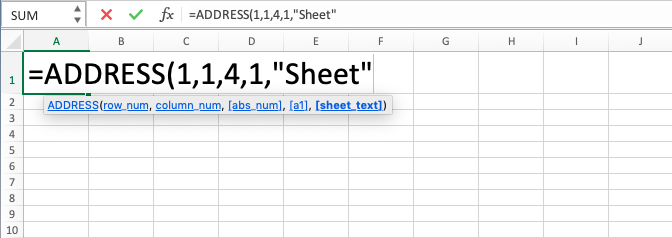ADDRESS Function in Excel - Compute Expert

Home >> Excel Tutorials from Compute Expert >> Excel Formulas List >> ADDRESS Function in Excel

In this tutorial, you will learn how to use ADDRESS formula in excel. ADDRESS function in excel can be used to get a cell coordinate with details that you need.

## Why do We Need to Learn About ADDRESS Formula in Excel?

In the data processing process in a spreadsheet, sometimes we need to get a cell coordinate as the input of a formula or as a hint where we can track the data that we need. For that, we need to specify the coordinate in the formula that we write or as the content of a cell so that it can be used as tracking for reference of a particular data when we need it.

As the example of this kind of usage, if we do formula writing for calculation (SUM, AVERAGE, PRODUCT, etc) or do an evaluation of the logic condition from a data, then often we need cell coordinates as the reference for the place of the numbers that want to be calculated or data reference for the logic condition to be evaluated. And in the case of data tracking reference, we want to write cell coordinates so other people later can find important data in the spreadsheet easily by looking at the cell coordinate reference that is written in the part of the spreadsheet.

In those cases, we should know about the positions of row and column or the name of the sheet where the data that we want to process or make a reference from is. But if there is a lot of data that needs to make the cell coordinates from, then, of course, it will be very troublesome if we do the writing of the cell coordinates manually.

For that purpose, you can use ADDRESS function in excel to help you. ADDRESS formula in excel can give cell coordinates that are needed as an input for a formula (by combining it with the INDIRECT also to help if you want the result of ADDRESS formula in excel to become an input of another formula) or as the content of a cell. It is very useful if you often need to refer to cell coordinates indirectly for your various needs.

## What is ADDRESS Function in Excel?

ADDRESS function in excel is a function with the usage objective to get the coordinate of a cell. This coordinate is later resulted in a text form and can be used as needed.

In general, the description of various inputs in ADDRESS formula in excel is as follows:

Notes:
• row_num = the row position of the cell coordinate
• col_num = the column position of the cell coordinate
• abs_num = optional. The number that determines whether you want the result to be absolute/not
• a1 = optional. The number that determines whether the type of the result is R1C1 or A1
• sheet_text = optional. The sheet name for the formula result later

## How to Use ADDRESS Formula in Excel?

The following part will explain in detail how to write ADDRESS function in excel. ADDRESS formula in excel needs required inputs of the row and column positions and optional inputs to communicate our desire whether the coordinate result is wanted to be absolute/not, R1C1/A1 type, and using what sheet name.

## Using ADDRESS Function in Excel

1. Type equal sign ( = ) in the cell where you want to put the result2. Type ADDRESS (can be with large and small letters) and open bracket sign after =3. Input the row position number as you desire and type a comma sign ( , )4. Input the column position number as you desire5. Optional: type a comma sign then input a number as the reference to whether you want the coordinate result to be absolute/not. The number options are 1 if you want the row and column to be absolute, 2 if you want absolute row and relative column, 3 if you want relative row and absolute column, 4 if you want relative row and column. If you don’t give input here, then the default is 16. Optional: type a comma sign then input a number as the reference for the type of the cell coordinate. The number options are 0 if you want the coordinate to have R1C1 form and 1 if you want the coordinate to have A1 form. If you don’t give input here, then the default is 17. Optional: type a comma sign then type the sheet name for the coordinate result later. If you don’t give input here, then the default is the currently active sheet (No sheet name at the result)8. Type close bracket sign9. Press Enter
10. The process of ADDRESS function in excel is done!## Exercise

After you have understood how to use ADDRESS formula in excel, now is the time you practice your understanding to sharpen it by doing the exercise of ADDRESS formula in excel below.

Download the exercise file of ADDRESS function in excel and do all the instructions. Please refer to the answer key file if you have done the exercise or confused about the result of the instructions!

### Instructions

1. Get the absolute, A1, and without sheet name cell coordinate of number 1!
2. Get the absolute column relative row, R1C1, and without sheet name cell coordinate of number 2!
3. Get the relative, A1, and with ‘3’ sheet name cell coordinate of number 3!

• Don’t forget to use INDIRECT if you want to use the result of this ADDRESS function in excel in another formula. If not, then the result of the formula will be an error
• You can also use ROW and COLUMN formulas to help you fill the inputs of row and number positions in the ADDRESS formula in excel!

Get updated excel info from Compute Expert by registering your email. It's free!